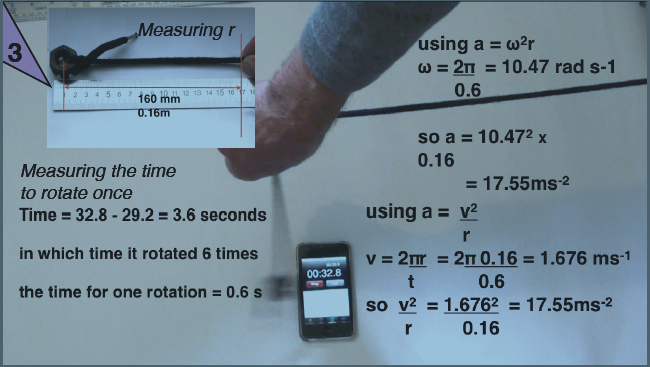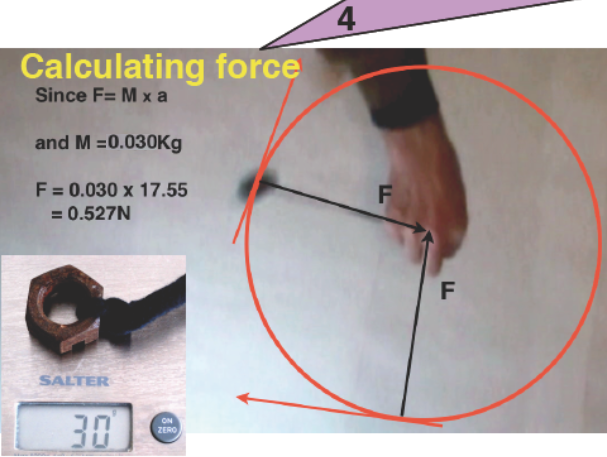Acceleration and forces in circular motion

When you swing something around in a circle on the end of a string it seems to be pulling outwards. Thinking about it more, you have to apply a force, you have to pull it in towards the centre. If you let go it flies off at a tangent.

Here there is only one force being applied, that's the one pulling it towards the centre. Therefore that must be an unbalanced force and the acceleration that causes must also be towards the centre.

Equations

The force needed to maintain circular motion depends upon three factors. These are the mass of the object being swung around, the speed that it is travelling at and the radius of the circle.

The equation we use is mv2/r    Where m is the mass of the object v is the speed in metres per second and r is the radius. We can alternatively use angular velocity.

Suppose we have a mass of 0.03 Kg rotating 6 times in 3.6 seconds around a circle of radius 0.16m then we can follow through the calculation here: